±а ј­ Бф СФ №ЬАнГЬВл: ЗлКдИл№ЬАнГЬВл, Ц»УР°жЦчІЕДЬ±ај­БфСФ. кЗіЖ: ЎЎ РФ±р: Л§ёз ГАЕ® І»ёжЛЯДг Email: АґЧФ: QQ: ЦчТі: РЕПўНј±к: ( Лж»ъ )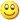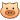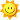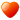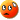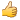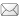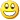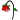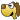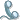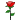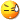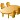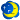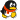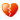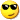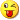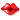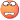BB ґъВл: ЧЦМе ЛОМе єЪМе БҐКй ї¬Ме УЧФІ Arial СХЙ« МмА¶ Ж·А¶ А¶Й« ЙоА¶ іИЙ« іИєм Йоєм ємЙ« Ч©єм °µєм ВМЙ« »ТВМ єЈВМ ·Ыєм №ыєм МТєм ЧПЙ« ЧПА¶ ЧШДѕ ЙієЦ НБ»Ж ЙоєЦ НБВМ »ТЙ« ґуРЎ 1єЕ 2єЕ 3єЕ 4єЕ 5єЕ БфСФёш(Л­?): ДЪИЭ: ёЗАПК¦ДъєГЈ¬ОЄКІГґФЪІйСЇµДК±єтµЪТ»ґОfetchµД cr=3,r=1?ГїґОІйСЇµДК±єтµЪТ»ґОfetchµД r = 1 ? SELECT * FROM EMP END OF STMT PARSE #1:c=0,e=435,p=0,cr=0,cu=0,mis=1,r=0,dep=0,og=4,tim=966751342 EXEC #1:c=0,e=35,p=0,cr=0,cu=0,mis=0,r=0,dep=0,og=4,tim=966753976 FETCH #1:c=0,e=47,p=0,cr=3,cu=0,mis=0,r=1,dep=0,og=4,tim=966754543 FETCH #1:c=0,e=66,p=0,cr=1,cu=0,mis=0,r=13,dep=0,og=4,tim=966755534